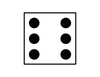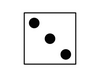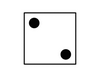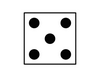## Petals Around the Rose

Here are five rolls of dice, with their score.

Roll #1 – Score: 6Roll #2 – Score: 4Roll #3 – Score: 4Roll #4 – Score: 8Roll #5: 0This is what you know:

1. The name of this game is significant (Petals Around the Rose)
2. The answer is always even

How is the score determined?

The solution is to count the dots (petals) surrounding the center dot (the rose).

Only odd numbers have a center dot, so even numbers (2, 4 and 6) are worth zero.

1 doesn’t have any surrounding dots, so it too is worth zero.

The three has two dots surrounding the center, so it’s worth two.

A five has four petals around the rose making it worth four.

Posted in Brain Teasers

4 Comments on "Petals Around the Rose"

Kouwpee says
December 14, 2016 @ 08:51

Interesting!

Leighve says
August 25, 2018 @ 23:04

I actually figured this one out. Quite proud of myself :D

Diego Arabit says
February 5, 2019 @ 23:17

The rules are false. My Computer engineering class was talking about how this puzzle is ambiguous when I came up with this proof; So the answer can be either an even number or zero. But this is where they are wrong. The answer can be zero, an even number or NOTHING, just non-existent. Anywho I hope everyone knows the method of finding answers here. (this can be Googled). So by now, we know that the middle dot represents the rose and the dots around represent the petals. So a roll of one has a rose with zero petals, a roll of 3 has a rose and 2 petals and a roll of 5 has a rose and 4 petals. All the others have no centre dot (rose) (but still have petals). So what happens if we roll, and a 3 or a 5 do not show up? Well, then the answer is zero because of the 1. But what if there is no 1? What if we roll and a 1, 3, or 5 do not show up? The answer cannot possibly be zero since the numbers 2, 4 and 6 do not have a rose in the middle. Yes, they have petals but they do not have the starting point of the flower. Therefore, the rules are false and in some cases, the answer is literally nothing. And when the answer is nothing the game can no longer be called petals around the rose because there is no rose.﻿ (also posted this on a YouTube comment as well)

Joycey says
January 10, 2020 @ 01:21

There is another way to solve this. Count up all the odd numbered dots and subtract from that total the number of dice that contain an odd number. For the first problem you add together 3+1+3+3 = 10. (and ignore the six The six because it’s an even number) Then you take 10 and subtract 4 from it, the number of dice that are odd and you get 6. This will work for all five dice sets including the last problem that is zero.